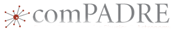# Determining Measured Values and UncertaintyThis material was originally developed through comPADRE
as part of its collaboration with the SERC Pedagogic Service.

## Summary

In this activity, students practice reading various measurement devices, such as graduated cylinders, electronic balances, voltmeters and spring scales. In each case students determine the range of possible values for the measurement. They express this range as a measured value with uncertainty in three forms, including a number line with error bars.

## Learning Goals

This activity is a building block in the Measurement and Uncertainty module. Students learn to determine the range of possible values for measurements using a variety of measurement tools, and to express that range numerically as absolute and relative uncertainty. Later activities teach students how to perform calculations using measurement values that include uncertainty.

## Description and Teaching Materials

There are three ways to conduct this activity: on paper, online, or in the lab. To do this activity on paper, students use photographs of the measurement devices, as in the worksheet linked below. Online, students view photos and videos of the measurement devices through a homework service such as WebAssign. Many photos and videos are provided below which can be used for either of these methods. Finally, stations can be set up in the lab and students take readings of the actual instruments. These methods can be used in concert. For example, the paper or online version can be used as practice or as an assessment after students have done the lab activity.

This worksheet can be used either for the activity itself, or as an assessment if the activity is done in the lab. worksheet for measurement and uncertainty activity (Microsoft Word 1.2MB Aug31 10)

No matter which method is used, the concepts are the same. Students must begin with the understanding that no measured value is ever exact; all measurements must be expressed as a range of values, that is, as a value with some uncertainty. Students use a measurement device to measure a quantity, with the goal in mind of determining the range of possible values within which the true value of the measurement lies. To find this range, students determine the highest possible value they think the measurement could be. That is, they determine the value below which they are certain the true value lies. Similarly, they determine the lowest possible value; the value above which they are certain the true value lies. Students then express these ranges as a measured value with uncertainty.

## Teaching Notes and Tips

If this activity is done in the lab, any measurement devices can be used. The instructor would need to set up various devices, such as thermometers, spring force gauges, electronic or mechanical balances or graduated cylinders.

If this activity is done on paper, the instructor can use the material provided here. worksheet for measurement and uncertainty activity (Microsoft Word 1.2MB Aug31 10)

If the activity is done online, the instructor may post the images provided in the file above, provided they are attributed to the author.

This activity can be completed in one class period. After students have determined the highest and lowest possible values, the rest of the work can be done outside of class.

## Assessment

As described above, the assessment for the lab-based version of this activity could be the paper version provided here.